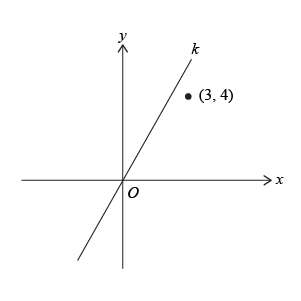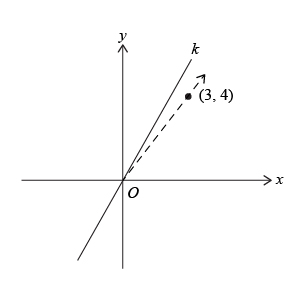# The slope of line k 1Quantity A \$\;\;\;\;\;\;\;\;\;\;\;\;\;\;\;\;\;\;\;\;\;\;\;\;\;\;\;\;\;\;\;\;\;\;\;\;\;\;\;\;\;\;\;\;\;\;\;\;\;\;\;\;\;\;\;\;\;\;\;\;\;\$ Quantity B \$\;\;\;\;\;\;\;\;\;\;\;\;\;\;\;\;\;\;\;\;\;\;\;\;\;\;\;\;\;\;\;\;\;\;\;\;\;\;\;\;\;\;\;\;\;\;\;\;\;\;\;\;\;\;\;\;\;\;\;\;\;\$ The slope of line \$k\$ \$\;\;\;\;\;\;\;\;\;\;\;\;\;\;\;\;\;\;\;\;\;\;\;\;\;\;\;\;\;\;\;\;\;\;\;\;\;\;\;\;\;\;\;\;\;\;\;\;\;\;\;\;\;\;\;\;\;\;\;\;\;\$ \$1\$
1. Quantity A is greater.
2. Quantity B is greater.
3. The two quantities are equal.
4. The relationship cannot be determined from the information given.

So, you were trying to be a good test taker and practice for the GRE with PowerPrep online. Buuuut then you had some questions about the quant section—specifically question 5 of Section 6 of Practice Test 1. Those questions testing our knowledge of Coordinate Geometry can be kind of tricky, but never fear, PrepScholar has got your back!

## Survey the Question

Let’s search the problem for clues as to what it will be testing, as this will help shift our minds to think about what type of math knowledge we’ll use to solve this question. Pay attention to any words that sound math-specific and anything special about what the numbers look like, and mark them on our paper.

We can see a graph of a line in the \$xy\$-plane, along with one of the quantities asking about the slope of a line. All of these are clues that we’ll be testing our Coordinate Geometry math skill here. Let’s keep what we’ve learned about this skill at the tip of our minds as we approach this question.

## What Do We Know?

Let’s carefully read through the question and make a list of the things that we know.

1. We have a graph of line \$k\$
2. Line \$k\$ goes through the origin \$(0,0)\$
3. Line \$k\$ goes above the point \$(3,4)\$
4. We want to compare the slope of line \$k\$ to the value \$1\$

## Develop a Plan

We’re asked for the slope of a line that is graphed, but we don’t have it’s equation. We do remember that we can find the slope of a line if we have two of its points. Specifically, if a line has point 1 \$(x_1,y_1)\$ and point 2 \$(x_2,y_2)\$, then the slope is the ratio of the difference in the \$y\$-values and the difference in the \$x\$-values:

\$\$\Slope = {y_2-y_1}/{x_2-x_1}\$\$

We can see that line \$k\$ goes through the origin, where the \$x\$-axis and \$y\$-axis intersect, so line \$k\$ has the point \$(0,0)\$ on it. While we don’t have a second point on line \$k\$, we can see that it has a greater slope than a line that would pass through the origin and point \$(3,4)\$. Let’s plan to draw in a line passing through the origin and point \$(3,4)\$, then see if that helps us narrow down the possible range of values for the slope of line \$k\$.

## Solve the Question

Let’s start by drawing in a dashed line passing through the origin and the point \$(3,4)\$.Let’s calculate the slope of this dashed line, using the points \$(0,0)\$ and \$(3,4)\$.

 \$\Slope\$ \$=\$ \${y_2-y_1}/{x_2-x_1}\$ \$ \$ \$ \$ \$\Slope\$ \$=\$ \${4-0}/{3-0}\$ \$ \$ \$ \$ \$\Slope\$ \$=\$ \$4/3\$

Visually we can see that line \$k\$ has a slope that is greater than the slope of the dashed line. Thus, line \$k\$ has a slope greater than \$4/3\$, which is greater than \$1\$. The correct answer is A, Quantity A is greater.

## What Did We Learn

We should definitely memorize how to calculate the slope of a line. We can think of it as “rise over run”, or the change in \$y\$ divided by the change in \$x\$.

\$\$\Slope = {y_2-y_1}/{x_2-x_1}\$\$

We also saw an interesting way to solve a Quantitative Comparison question here. Although we could not directly calculate the slope of line \$k\$, we could calculate the slope of another line, and then rationalize that the slope of line \$k\$ must be greater than the slope of that other line. Very clever of us indeed.

Want more expert GRE prep? Sign up for the five-day free trial of our PrepScholar GRE Online Prep Program to access your personalized study plan with 90 interactive lessons and over 1600 GRE questions.